# Baire classes

(diff) ← Older revision | Latest revision (diff) | Newer revision → (diff)
Families of real functions which are defined inductively using the ordinal number of limit transitions involved in the definition of the function, and which constitute the classification of functions proposed in 1899 by R. Baire , known as the Baire classification. The set of all continuous functions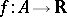, whereis a metric space, is called the zero-th Baire class. The first Baire class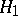is the set of discontinuous functionswhich are the limit of a sequence of continuous functions converging at each point. The Baire class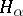, whereis an ordinal number of the first or second class, is defined as the set of functionswhich do not form part of any of the preceding classes, but which may be represented as, where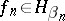,. The union of the Baire classesover all ordinal numbers of the first and second class constitutes the set of Baire functions. This is the minimal class of functionscontaining all the continuous functions and closed with respect to pointwise convergence. A linear combination, product and quotient (for non-zero denominators) of functions of a Baire class not higher thanis a function in a Baire class not higher than. A uniformly convergent sequence of functions of a Baire class not higher thanhas as limit a function in a Baire class not higher than. Necessary and sufficient conditions have been established for a sequence of functions in a Baire class not higher thanto converge to a function in a Baire class not higher than. The union of all dense-in-itself sets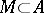is called the kernel of the topological space. Ifis a complete space with a non-empty dense-in-itself kernel, none of the Baire classes is empty . The set of Baire functions coincides with the set of Borel-measurable functions (cf. Borel measure), and for this reason they are all Lebesgue-measurable (cf. Lebesgue measure). A Lebesgue-measurable function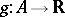is equivalent to a Baire function of a Baire class not higher than two . Baire, who considered functions defined on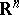(mainly on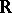), made the most detailed study of functions of the first class. He showed that a necessary and sufficient condition for a discontinuous function to belong to the first class is the existence of a point of continuity of the induced function on each perfect set (Baire's theorem). This theorem is applicable to a functionifhas the Baire property . The concept of a Baire function can be naturally generalized to functions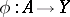, where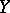is an arbitrary metric space.